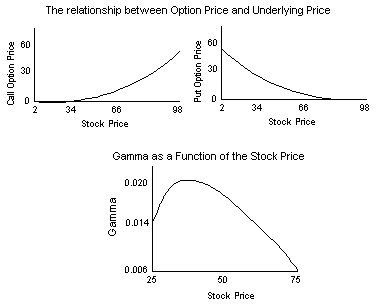### Why should I choose AnalystNotes?

Simply put: AnalystNotes offers the best value and the best product available to help you pass your exams.

### Subject 8. The gamma effect on an option's price and delta

Unlike the other sensitivity measures such as Delta, RHO, Theta or Vega, Gamma does not measure the sensitivity of the price of an option to one of the parameters of the Black-Scholes-Merton model. Instead, it measures how Delta changes with changes in the stock price. In terms of calculus, Gamma is the second derivative of the option price with respect to the stock price.

The following exhibit shows the relationship between the option price and the underlying stock price, and the relationship between the Gamma and the underlying stock price. (X = 50, r(c) = 0.0488, T = 0.75, σ = 0.35).• Call option values increase the greater the underlying value, and put option values decrease. However, the amount of change is not the same in each direction. N(d1) measures the slope of this line at a given point. As such, it measures only the slope for a very small change in the underlying.

• When the underlying changes by more than a very small amount, the curvature of the line comes into play and distorts the relationship between the option price and the underlying price that is explained by delta.

• The curvature or second-order effect is known as the gamma in the options world (convexity in the fixed income world). When gamma is large, the delta changes rapidly and cannot provide a good approximation of how much the option moves for each unit of movement in the underlying.

• The "Gamma as a Function of the Stock Price" figure applies to both the put and call option, because the Gammas are the same for a put and call with the same underlying instrument, time to expiration, and strike price.

• Gamma is large when the option is near-the-money. However, it is not necessarily the largest when the option is at-the-money (in the above graph: when the underlying is at 50), as it is affected by other factors as well. Gamma is small when the option is deep-in-the-money or deep-out-of-the-money.

• Gamma also varies with the time remaining until expiration. For an option that is near-the-money, gamma increases as expiration approaches. However, it is difficult to make solid generalizations about how gamma will change without actually calculating the effects of the passage of the time.

#### Practice Question 1

When gamma is large,

A. the underlying price must be very large.
B. the option price must be very large.
C. for a small change in underlying price, there will be a large change in option price.
D. none of them is correct.

Gamma measures how sensitive the delta is to a change in the underlying.

#### Practice Question 2

I. For a call option, gamma increases the greater the underlying value.

II. For a call option, delta increases the greater the underlying value.

For a call option, option price increases the greater the underlying value.

#### Practice Question 3

Gamma is small when the option is

I. near-the-money.
II. at-the-money.
III. deep-in-the-money.
IV. deep-out-of-the-money.

Gamma is large when the option is near-the-money (or close to at-the-money).
Gamma is small when the option is deep-in-the-money or deep-out-of-the-money.

#### Practice Question 4

When gamma is large,

A. there will be a large change in the option price for a small change in the underlying price.
B. there will be a large change in the underlying price for a small change in the option price.
C. there will be a large change in the change rate of the option price for a small change in the underlying price.

Gamma measures how sensitive the delta is to a change in the underlying, and delta measures the change rate of the option price for a small change in the underlying price.

#### Practice Question 5

Which of the following statements is (are) true with respect to option Gammas?

I. For a call and a put that have the exact same parameters, their gammas will be equal.
II. For a call that's deep in-the-money, its gamma will be very high.
III. For a put that's deep out-of-money, its gamma will be very low.
IV. The gamma for both a long put and a long call option can only be positive.

A. I and III
B. I and II
C. II, III and IV